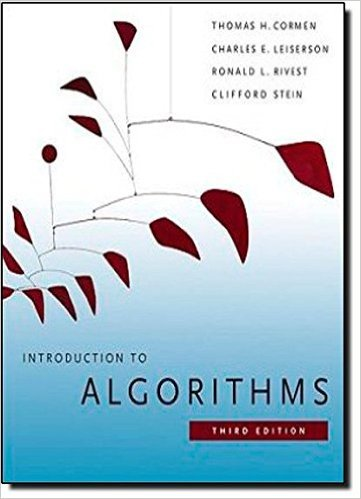×
Get Full Access to Introduction To Algorithms - 3 Edition - Chapter 12 - Problem 12-3
Get Full Access to Introduction To Algorithms - 3 Edition - Chapter 12 - Problem 12-3

×

# Average node depth in a randomly built binary search treeISBN: 9780262033848 130

## Solution for problem 12-3 Chapter 12

Introduction to Algorithms | 3rd Edition

• Textbook Solutions
• 2901 Step-by-step solutions solved by professors and subject experts
• Get 24/7 help from StudySoup virtual teaching assistantsIntroduction to Algorithms | 3rd Edition

4 5 1 412 Reviews
18
2
Problem 12-3

Average node depth in a randomly built binary search tree In this problem, we prove that the average depth of a node in a randomly built binary search tree with n nodes is O.lg n/. Although this result is weaker than that of Theorem 12.4, the technique we shall use reveals a surprising similarity between the building of a binary search tree and the execution of RANDOMIZEDQUICKSORT from Section 7.3. We define the total path length P .T / of a binary tree T as the sum, over all nodes x in T , of the depth of node x, which we denote by d.x; T /. for Chapter 12 305 011 0 100 10 1011 0 1 1 0 1 01 1 Figure 12.5 A radix tree storing the bit strings 1011, 10, 011, 100, and 0. We can determine each nodes key by traversing the simple path from the root to that node. There is no need, therefore, to store the keys in the nodes; the keys appear here for illustrative purposes only. Nodes are heavily shaded if the keys corresponding to them are not in the tree; such nodes are present only to establish a path to other nodes. a. Argue that the average depth of a node in T is 1 n X x2T d.x; T / D 1 n P .T / : Thus, we wish to show that the expected value of P .T / is O.n lg n/. b. Let TL and TR denote the left and right subtrees of tree T , respectively. Argue that if T has n nodes, then P .T / D P .TL/ C P .TR/ C n 1 : c. Let P .n/ denote the average total path length of a randomly built binary search tree with n nodes. Show that P .n/ D 1 n Xn1 iD0 .P .i / C P .n i 1/ C n 1/ : d. Show how to rewrite P .n/ as P .n/ D 2 n Xn1 kD1 P .k/ C .n/ : e. Recalling the alternative analysis of the randomized version of quicksort given in 7-3, conclude that P .n/ D O.n lg n/. 306 Chapter 12 Binary Search Trees At each recursive invocation of quicksort, we choose a random pivot element to partition the set of elements being sorted. Each node of a binary search tree partitions the set of elements that fall into the subtree rooted at that node. f. Describe an implementation of quicksort in which the comparisons to sort a set of elements are exactly the same as the comparisons to insert the elements into a binary search tree. (The order in which comparisons are made may differ, but the same comparisons must occur.)

Step-by-Step Solution:
Step 1 of 3

L23 - 4 How can we ﬁnd those extreme values Def. Aufcin f has a local (relative) maximum at c if there is an open interval I containing c such that for all x in I Def. Au fcin f has a local (relative) minimum at c if there is an open interval I containing c such that for all x in I ex. Find all local and absolute extrema of the function f(x)skhdb l. abc e d

Step 2 of 3

Step 3 of 3

##### ISBN: 9780262033848

Unlock Textbook Solution